×#### Thank you for registering.

One of our academic counsellors will contact you within 1 working day.

Click to Chat

1800-1023-196

+91-120-4616500

CART 0

• 0

MY CART (5)

Use Coupon: CART20 and get 20% off on all online Study Material

ITEM
DETAILS
MRP
DISCOUNT
FINAL PRICE
Total Price: Rs.

There are no items in this cart.
Continue Shopping• Complete JEE Main/Advanced Course and Test Series
• OFFERED PRICE: Rs. 15,900
• View Details

```Chapter 32: Statistics – Exercise 32.4

Statistics – Exercise – 32.4 – Q.1(i)

x
d = (x - Mean)
d2

2
-5
25

4
-3
9

5
-2
4

6
-1
1

8
1
1

17
10
100

42

140Statistics – Exercise – 32.4 – Q.1(ii)

x
d = (x - Mean)
d2

6
-3
9

7
-2
4

10
1
1

12
3
9

13
4
16

4
-5
25

8
-1
1

12
3
9

72

74Statistics – Exercise – 32.4 – Q.1(iii)

xi
di = xi - 299
d12

227
-72
5184

235
-64
4096

255
-44
1936

269
-30
900

292
-7
49

299
0
0

312
13
169

321
22
484

333
34
1156

348
49
2401

Total = – 99
Total = 16375Statistics – Exercise – 32.4 – Q.1(iv)

xi
di = xi - 299
d12

15
0
0

22
7
49

27
12
144

11
-4
16

9
-6
36

21
6
36

14
-1
1

9
-6
36

Total = – 8
Total = 318Statistics – Exercise – 32.4 – Q.2

We have, n = 20, and σ2 = 5

Now each observation is multiplied by 2.

Suppose X = 2x be the new data.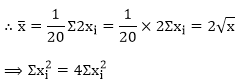Since, σ2 = 5Now, for the new data:Statistics – Exercise – 32.4 – Q.3

We have, n = 15, and σ2 = 4

Now each observation is increased by 9.

Suppose x = x + 9 be the new data.Since, σ2 = 5Now, for the new data:Statistics – Exercise – 32.4 – Q.4

Let the other two be x and y

1 + 2 + 6 + x + y = 5 * 4.4 because of the mean

x + y = 13

Variance = [(1 - 4.4)2 + (2 - 4.4)2 + (6 - 4.4)2 + (x - 4.4)2 + (y - 4.4)2]/5

Hence

11.56 + 5.76 + 2.56 + (x - 4.4)2 + (y - 4.4)2 = 41.2

(x - 4.4)2 + (13 - x - 4.4)2 = 21.32

x2 - 8.8x + 19.36 + 73.96 - 17.2x + x2 = 21.32

2x2 - 26x + 72 = 0

x2 - 13x + 36 = 0

(x - 4)(x - 9) = 0

x = 4 or x = 9

If x = 4, y = 9 and

The other two observations are 4 and 9.

Statistics – Exercise – 32.4 – Q.5

If mean and SD of observations areand σ respectively, then mean and SD of observations multiplied by a constant 'k' are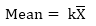SD = |k|σ

In this question, it is given that k = 3

So New mean = 8 × 3 = 24

New SD = 4 × 3 = 12

Statistics – Exercise – 32.4 – Q.6

Let x and y be the remaining two observations. Then,

Mean = 9⟹ 60 + x + y + = 72

⟹ x + y = 12 ..... (i)

Variance = 9.25⟹ 642 + x2 + y2 = 722

⟹ x2 + y2 = 80 ..... (ii)

Now, (x + y)2 + (x - y)2 = 2(x2 + y2)

⟹ 144 + (x - y)2 = 2 × 80

⟹ (x - y)2 = 16

⟹ x - y = ±4

if x - y  = 4, then x + y = 12 and x - y = 4 ⟹ x = 8, y = 4

if x - y = -4, then x + y = 12 and x - y = -4 ⟹ x = 4, y = 8

Hence, the remaining two observations are 4 and 8.

Statistics – Exercise – 32.4 – Q.7

We have,

n = 200,= 40, σ = 15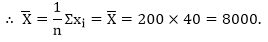Corrected Σxi = Incorrect Σxi - (Sum of incorrect values) + (Sum of correct values)

= 8000 - 34 - 53 + 43 + 35 = 7991= 365000 - (34)2 - 532 + (43)2 + 352 = 364109

891Statistics – Exercise – 32.4 – Q.8

We have,

n = 100,= 40, σ = 5.1Corrected Σxi = incorrect Σxi - (Sum of incorrect values) + (Sum of correct values)

= 4000 - 50 + 40 = 3990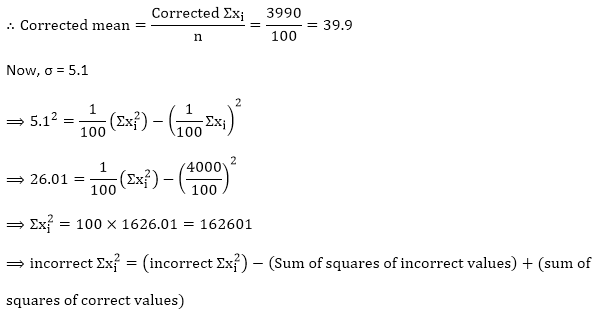= 162601 - (50)2 + (40)2 = 161701Statistics – Exercise – 32.4 – Q.9

We have,

n = 20,= 10 and σ = 2⟹ Incorrected Σxi = 200

and,(i) When 8 is omitted from the data:

If 8 is omitted from the data, then 19 observations are left.

Now, Incorrected Σxi = 200

⟹ Corrected Σxi + 8 = 200

⟹ Corrected Σxi = 192

and,(ii) When the incorrect observation 8 is replaced by 12:

we have, Incorrected Σxi = 200

∴ Corrected Σxi = 200 - 8 + 12 = 204

and,Statistics – Exercise – 32.4 – Q.10

We have,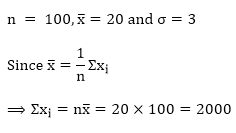⟹ Incorrect Σxi = 2000

and,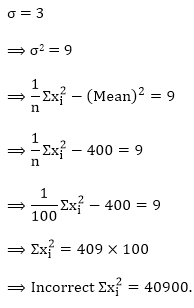When the incorrect observations 21, 21, 18 are omitted from the data:

n = 97

Now, Incorrect Σxi = 2000

⟹ Corrected Σxi = 2000 - 21 - 21 - 18 = 1940

and,Statistics – Exercise – 32.4 – Q.11

We have,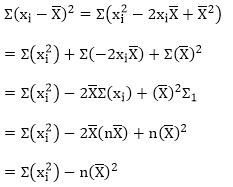Dividing both the sides by n we get,Taking square root on both the sides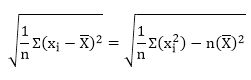```### Course Features

• 728 Video Lectures
• Revision Notes
• Previous Year Papers
• Mind Map
• Study Planner
• NCERT Solutions
• Discussion Forum
• Test paper with Video Solution